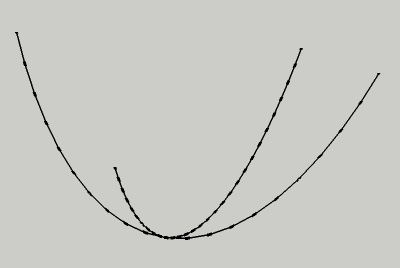Engineering ToolBox - Resources, Tools and Basic Information for Engineering and Design of Technical Applications!

# Solving Quadratic Equation with One Unknown

## How to solve a quadratic equation.

The quadratic equation can be expressed as

a x2 + b x + c = 0                      (1)

which can be solved for the two alternatives of x as

x1,2 = (-b ± (b2 - 4 a c)1/2) / (2 a)             (2)

### Example - Solving Quadratic Equation

If

a = 1

b = 6

c = 5

then the two alternatives for x can be calculated as

x1 = (-6 + (62 - 4 1 5)1/2) / (2 1)

= -1

x2 = (-6 - (62 - 4 1 5)1/2) / (2 1)

= -5## Related Topics

• Mathematics - Mathematical rules and laws - numbers, areas, volumes, exponents, trigonometric functions and more.

## Engineering ToolBox - SketchUp Extension - Online 3D modeling!

Add standard and customized parametric components - like flange beams, lumbers, piping, stairs and more - to your Sketchup model with the Engineering ToolBox - SketchUp Extension - enabled for use with the amazing, fun and free SketchUp Make and SketchUp Pro .Add the Engineering ToolBox extension to your SketchUp from the SketchUp Pro Sketchup Extension Warehouse!

Translate

## Privacy

We don't collect information from our users. Only emails and answers are saved in our archive. Cookies are only used in the browser to improve user experience.

Some of our calculators and applications let you save application data to your local computer. These applications will - due to browser restrictions - send data between your browser and our server. We don't save this data.

## Citation

• Engineering ToolBox, (2022). Solving Quadratic Equation with One Unknown. [online] Available at: https://www.engineeringtoolbox.com/quadratic-equation-one-unknown-d_2209.html [Accessed Day Mo. Year].

Modify access date.

. .

#### Scientific Online Calculator3 30